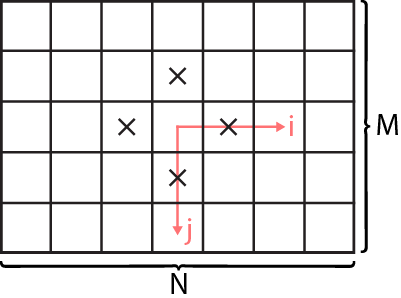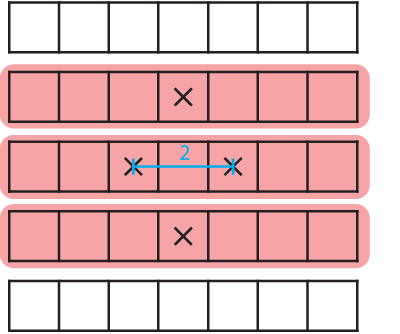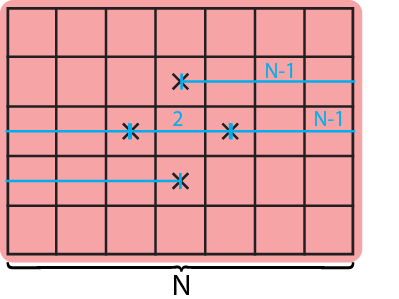# Layer Conditions

We will briefly explain what layer conditions are, give a detailed example and provide a tool for their interactive evaluation. This will help you understand and calculate optimal blocking sizes for stencil codes.

Layer conditions (LCs) allow a quick approximation of the cache requirement of stencil codes. The model assumes a perfect least-recently-used (LRU) cache replacement policy, which has proven to give a good estimate on current architectures with a pseudo LRU caching policy (e.g., current Intel and AMD CPUs).

The two-dimensional layer condition (the most relevant for stencil in 2D) is given by multiplying the stencil height ($$S_h [\mathrm{elements}]$$) with the matrix (or array) width ($$M_w [\mathrm{elements}]$$) and the size per element ($$s [\frac{bytes}{\mathrm{element}}]$$), if that fits into applicable cache size ($$C_a [\mathrm{bytes}]$$) the layer condition is fulfilled and all accesses in two dimensions are reduced to one cache miss: $S_h*M_w*s\leq C_a$

Consider a 2D 5-Point stencil (explained in more detail in next section) on a 1024x1024 matrix with single precision floating point numbers: the stencil has a height of three rows ($$S_h=3\,\mathrm{elements}$$), the matrix has a width of 1024 columns ($$M_w=1024\,\mathrm{elements}$$) and a single precision floating point number takes four bytes ($$s=4\,\frac{\mathrm{bytes}}{\mathrm{element}}$$). A typical code will use two arrays, one for input and one for output, and swap them after each sweep. Therefore the applicable cache size is half of the actual cache size ($$C_a = \frac{C_s}{2}\,\mathrm{bytes}$$): $(3*1024*4)\,\mathrm{bytes}\leq \frac{C_s}{2}$

Any cache larger than 24 kilobyte fulfills the condition and thus leads to cache hits to all but two elements (leading elements from input matrix and output matrix). This example can also be calculated with our interactive tool: load example. The approach explained here only works if the stencil is without row gaps. To overcome this problem we propose a more detailed analysis explained in the following section.

A simple example to explain stencil codes is the 2D 5-Point stencil kernel, which is a common stencil that results from a finite difference discretization. The code will look something like in the following block.

As you can see, it iterates over the inner elements of an MxN array, reads four elements from a and writes back one element into array b. While doing so it does some additions and a multiplication with a scalar, which we will ignore since arithmetical operations do not affect the cache behavior. Important are the array accesses b[j][i], a[j+1][i], a[j][i-1], a[j][i+1], a[j-1][i] and the array definition a[M][N] and b[M][N], the combination of these dictate the cache requirement of this code.

To understand the behavior we need to know how the arrays are serialized in memory and what caches do to speed up memory accesses:

In C, multidimensional arrays are stored in the row-major order, which stores consecutive elements in rows in consecutive memory locations: e.g., a[j][i] and a[j][i+1] will be located next to one another in memory. Thus, a[j][i] and a[j+1][i] will be far apart, as far as the inner dimension is long (in the example $$N$$). For the arrays in the example, the following two memory addresses are equivalent: &a[j][i] and a+j*N+i.

Caches speed up subsequent memory accesses to the same location by retaining already accessed data in small–but fast–caches. This is done transparently and requires no special instructions to utilize. On current CPUs typically three cache-levels are found, L1 to L3, with increasing size and latency, and decreasing bandwidth. If a data can be served from a cache it is considered a cache hit, otherwise it is a cache miss and will be passed onto the next cache level or main memory. On each miss, the missed data will be pulled into the cache for further use. This means that caches are always full and some data needs to be evicted to free up space, which data is removed is decided by the replacement policy, which is typically some form of least-recently-used (LRU). LRU always removes the data which has not been used for the longest period.

double a[M][N];
double b[M][N];
double s = 1.0/4.0;

for(int j=1; j<M-1; j++) {
for(int i=1; i<N-1; i++) {
b[j][i] = s * (
a[j-1][i]
+ a[j][i-1] + a[j][i+1]
+ a[j+1][i]);
}
}

If we plot these accesses onto a small array (here 5x7), it looks as seen in the graphic. Each X marks a read access to array a and the iteration order is denoted by the arrows i and j. By iterating in direction of i and j, we see that there will be a significant reuse of elements happening. Each element will be touched four times (if we ignore the boundary values). The number of iterations between the subsequent touches is what will influence the cache requirement most, due to the assumed LRU replacement policy.

It is important to note that the order of the for-loops and the order of indices found in the array access must be the same for this model to work. E.g., an access a[i][j] would not be suitable in the code.## 1D Layer Condition

We will build the layer condition model starting from the first dimension, which may seem tedious or useless–since a 1D layer condition is almost always fulfilled and considered trivial–but it allows for a better understanding and will enable the reader to easily project this model to any higher dimension.

First we slice our array access into one dimensional continuous segments. This will yield three slices for the array a, as marked red in the graphic. Since b has only one access, it will always yield only one slice, no matter the dimension. We need to keep the total number of slices in mind for later computation:

$n_\mathrm{slices}=3+1=4$

In addition we need to compute the relative offsets of accesses within each slice. In this case it is easy, because there is only one slice containing more then one accesses: the center row with a[j][i-1] and a[j][i+1]. They are offset by two elements. We need to keep a list of offsets, to which we add this:

$L_\mathrm{rel.offsets}=\{2\}$Now we have everything we need to make a cache requirement estimation: the number of slices, the relative offsets and the size of an element. The latter we know from the array definition, which was double and has eight bytes per element ($$s=8\frac{\mathrm{byte}}{\mathrm{element}}$$).

The total cache requirement is the sum over all the relative offsets and the maximum over all relative offsets times the number of slices: $C_\mathrm{req.}=\left(\sum L_\mathrm{rel.offsets}+\max(L_\mathrm{rel.offsets})*n_\mathrm{slices}\right)*s$

In our case this will turn out to be $$C_\mathrm{req.}=(2+2*4)*8=80\,\mathrm{Byte}$$, which is ridiculously small and will fit in any cache. As long as $$C_\mathrm{req.}$$ is smaller then half the cache, we will reuse at least one (number of entries in $$L_\mathrm{rel.offsets}$$) element.

## 2D Layer Condition

We will now evolve the previous model to two dimensions, which will give us a more useful estimation. This will be the highest dimension analyzable, since the arrays are two dimensional.

Again, we slice our array access into two dimensional continuous segments. This is easy, since the array only has two dimensions, therefore we have only one slice per array (marked red in graphic). One for a and another for b:

$n_\mathrm{slices}=1+1=2$

To calculate the relative offsets between accesses within each slice is a little more laborious. We have four accesses in a, thus three relative offsets to add to our list: $$N-1$$ elements between a[j-1][i] and a[j][i-1], $$2$$ elements between a[j][i-1] and a[j][i+1] (as in the 1D case), and again $$N-1$$ elements a[j+1][i] and a[j][i+1].

$L_\mathrm{rel.offsets}=\{N-1, 2, N-1\}$Putting it all together follows the same process as before. We take the number of slices $$n_\mathrm{slices}$$, the relative offsets $$L_\mathrm{rel.offsets}$$ and $$s=8\frac{\mathrm{byte}}{\mathrm{element}}$$, the size of an element and we get an estimation of the total cache requirement by: $C_\mathrm{req.}=\left(\sum L_\mathrm{rel.offsets}+\max(L_\mathrm{rel.offsets})*n_\mathrm{slices}\right)*s$

More interesting is what results from it: $$C_\mathrm{req.}(N)=(2*N+(N-1)*2)*8=32*N-16\,\mathrm{Byte}$$, which–unlike before–depends on $$N$$. This means that aslong as $$C_\mathrm{req.}(N)$$ is smaller then half the cache, three out of five data accesses are served from cache.

The optimal blocking size is calculated by solving the above equation for N, such that it is equal to half the cache-size. Since this model is assuming a perfect cache an no sharing of resources, we usually incorporate a safety margin of two, which means that we use the prediction for half the cache size. To fit into a 32 KB cache we take: $N=\frac{\frac{1}{2}*32*1024+16}{32}\approx512$ Thus, the optimal blocking size would be 512 elements in the inner-most loop.

How many dimensions does your code have? The number of nested for-loops AND array dimensions (e.g. a[][] is of dimension 2).

 double double _Complex float float _Complex

Number and size of elements. All arrays have to be of same size.

Add accesses found in the innermost loop without the loop counters. E.g. a[+1][+1] or VEC[-1]. If writes go to the cache, also add write accesses here.

KB
core(s)
KB
core(s)
KB
core(s)

Sizes of data caches (all are assumed inclusive with least-recently-used caching). Sharing means that the available cache size will be divided by the number of cores. For SMT-2, set sharing of L1 and L2 to 2 cores. For 4 threads with SMT-1, set L3 (usually shared by all cores) to 3 cores, and set L1 and L2 to 1 core.

Blocking prediction will be reduced by this factor.

Author: Julian Hammer, licensed under AGPLv3.

Get in touch with our group: hpc@rrze.fau.de